# 5 ways to sum a column in Excel 2016 - 2010

This tutorial shows how to sum a column in Excel 2010 - 2016. Try out 5 different ways to total columns: find the sum of the selected cells on the Status bar, use AutoSum in Excel to sum all or only filtered cells, employ the SUM function or convert your range to Table for easy calculations.

If you store such data as price lists or expense sheets in Excel, you may need a quick way to sum up prices or amounts. Today I'll show you how to easily total columns in Excel. In this article, you'll find tips that work for summing up the entire column as well as hints allowing to sum only filtered cells in Excel.

Below you can see 5 different suggestions showing how to sum a column in Excel. You can do this with the help of the Excel SUM and AutoSum options, you can use Subtotal or turn your range of cells into Excel Table which will open new ways of processing your data.

### How to sum a column in Excel with one click

There is one really fast option. Just click on the letter of the column with the numbers you want to sum and look at the Excel Status bar to see the total of the selected cells.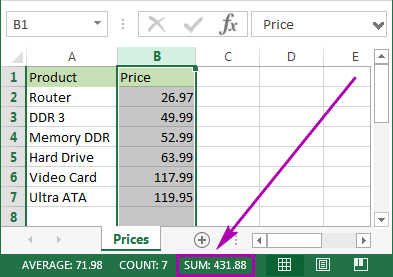Being really quick, this method neither allows copying nor displays numeric digits.

## How to total columns in Excel with AutoSum

If you want to sum up a column in Excel and keep the result in your table, you can employ the AutoSum function. It will automatically add up the numbers and will show the total in the cell you select.

1. To avoid any additional actions like range selection, click on the first empty cell below the column you need to sum.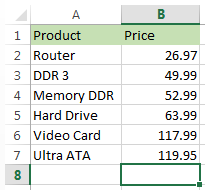• Navigate to the Home tab -> Editing group and click on the AutoSum button.• You will see Excel automatically add the =SUM function and pick the range with your numbers.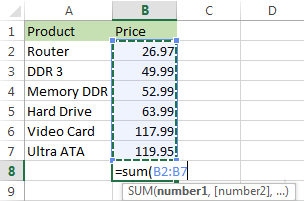• Just press Enter on your keyboard to see the column totaled in Excel.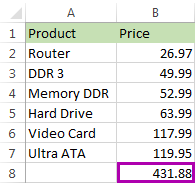This method is fast and lets you automatically get and keep the summing result in your table.

## Enter the SUM function manually to sum a column In Excel

You can also enter the SUM function manually. Why would you need this? To total only some of the cells in a column or to specify an address for a large range instead of selecting it manually.

1. Click on the cell in your table where you want to see the total of the selected cells.2. Enter `=sum(` to this selected cell.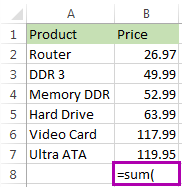3. Now select the range with the numbers you want to total and press Enter on your keyboard.
Tip. You can enter the range address manually like `=sum(B1:B2000)`. It's helpful if you have large ranges for calculation.That's it! You will see the column summed. The total will appear in the correct cell.This option is really handy if you have a large column to sum in Excel and don't want to highlight the range. However, you still need to enter the function manually. In addition, please be prepared that the SUM function will work even with the values from hidden and filtered rows. If you want to sum visible cells only, read on and learn how.

## Use Subtotal in Excel to sum only filtered cells

This feature is perfect for totaling only the visible cells. As a rule, these are filtered or hidden cells.

1. First, filter your table. Click on any cell within your data, go to the Data tab and click on the Filter icon.2. You will see arrows appear in the column headers. Click on the arrow next to the correct header to narrow down the data.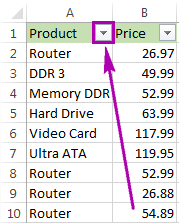3. Uncheck Select All and tick off only the value(s) to filter by. Click OK to see the results.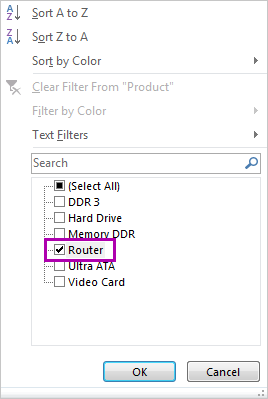4. Select the range with the numbers to add up and click AutoSum under the Home tab.Voila! Only the filtered cells in the column are summed up.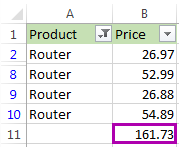If you want to sum visible cells but don't need the total to be pasted to your table, you can select the range and see the sum of the selected cells on the Excel Status bar. Or you can go ahead and see one more option for summing only filtered cells.

## Convert your data into Excel table to get total for your column

If you often need to sum columns, you can convert your spreadsheet to Excel Table. This will simplify totaling columns and rows as well as performing many other operations with your list.

1. Press Ctrl + T on yourkeyboardto format the range of cells as Excel Table.
2. You will see the new Design tab appear. Navigate to this tab and tick the checkbox Total Row.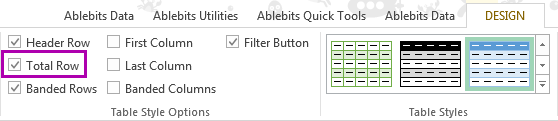3. A new row will be added at the end of your table. To make sure you get the sum, select the number in the new row and click on the small down arrow next to it. Pick the Sum option from the list.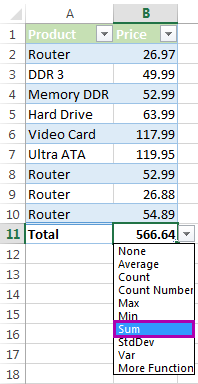Using this option lets you easily display totals for each column. You can see sum as well as many other functions like Average, Min and Max.

This feature adds up only visible (filtered) cells. If you need to calculate all data, feel free to employ instructions from How to total columns in Excel with AutoSum and Enter the SUM function manually to total the column.

Whether you need to sum the entire column in Excel or total only visible cells, in this article I covered all possible solutions. Choose an option that will work for your table: check the sum on the Excel Status bar, use the SUM or SUBTOTAL function, check out the AutoSum functionality or format your data as Table.

If you have any questions or difficulties, don't hesitate to leave comments. Be happy and excel in Excel!

### 71 responses to "5 ways to sum a column in Excel 2016 - 2010"

1.Amanda says:

Hi, Svetlana,

I'm a bit stuck on finding a formula that will suit my needs. My spreadsheet has column A: first name; column B: last name; column C: type of training; column D: date of training.

I need a formula that will look at column A, B, and C and if they match any other row in column A, B, and C I need it to select the most recent date.

this will remove all duplicate training and keep only their most current training record.

Any suggestions? i'm stumped on this one.

2.Ryan says:

Amanda,

The semi-manual approach to assist manual deletion would be to use Auto Filter or Sort to display sorted lists sorted by column C, then column B (where C is the same) then column A (where the previous two are the same), then column D (where all are the same). This way you get a block of rows with Jane, Smith, Excel Training Basics, Date and can keep the latest date and delete the rest.

The automated way would be to insert a new Worksheet to show the latest training only, leaving previous training on your existing Worksheet, say "Sheet1". You could then write a Visual Basic macro to copy rows from "Sheet1" into your new sheet, sort them and find the most recent dates of each instance where A, B, and C match up. Recording a macro where you manually invoke the appropriate Copy and Sort will help you see which functions to call to automate it, but it's hardly a beginner's task.

•Maria Azbel (Ablebits.com Team) says:

Thank you for your help, Ryan!

•meshack says:

Congratulations to you, am former. computer study I enjoyed the program of ISU

3.Raghu Setty says:

when I insert data into columns and press auto sum, i get the total. But when I delete the data from one of the column, the total still remains the same. It doesn't change. How do i rectify this problem?

4.Rawa says:

Hi, Is there any way to change for example =4+4+5+6 to column like
4
4
5
6

Regards

•Maria Azbel (Ablebits.com Team) says:

Hi Rawa,

First you need to use the Excel Text to Columns option to get the numbers in separate cells. Then use Paste Special -> Transpose.

5.francis says:

How can you get the AutoSum of all fill column blocks.

6.arman says:

بسم الله الرّحمن الرّحیم
hello
thank you very much.
It,s very good....

7.Alberta says:

I want every cell in the row more than 60 to give me the difference otherwise if it is 60 than give me a 0
My formula looks like:
=IF('TOTAL WEEK 1'!\$D\$4:\$R\$4>60;'TOTAL WEEK 1'!\$D\$4:\$R\$4-60;0)
But if give me only a result if it is more in the first cell not in the following cells .

8.naponica skannal says:

Good Afternoon, I wanted to know how do I capture all the total in line C.To add all totals together. I know I click on each total with the plus sign. It's just not working can you tell me what other keys I need to hold. Thanks

9.hemanth says:

i want to count total 10 cell in single short i am using sum formula, my problem is in between 2 cell have a formula that 2 cell values not count in total how to count 10 cell

10.Michele says:

Is there a way to total the sums of only the highlighted cells in a spreadsheet? I accrue my expenses for each of my jobs and then highlight when the expense is received so it would be nice to be able to determine what expenses have been received already.

•Renat Tlebaldziyeu (Ablebits.com Team) says:

Hi Michele,

11.pavithra says:

how to do outstanding amount. example: 1+1+1 total = 3 outstanding 0

•Renat Tlebaldziyeu (Ablebits.com Team) says:

Hi pavithra,

12.Sugeetha says:

if i want get a sum of cells data in a column (if it is in time format like 7:30, 1:45 what is the method please ?

if i do it normal way it calculates up to 24:00 and total is a wrong figure ?

13.Dragan says:

kako u excelu dobijene vrednosti zaokruziti na 5 ili 9
npr. 27,2 =25 ili 27,6 =29

14.benito says:

kako u excelu dobijene vrednosti zaokruziti na 5 ili 9
npr. 27,2 =25 ili 27,6 =29

•Renat Tlebaldziyeu (Ablebits.com Team) says:

Hi benito,

You should use the following formula:
=IF(MOD(A1, 5) >= 2.5, QUOTIENT(A1, 5) * 5 + 4, QUOTIENT(A1, 5) * 5)

15.rupesh Bhaje says:

hiii

i have to sum

1200 cft (i have to mention amount & Measurment both in each sales )
1300 cft
1400 cft
total---------?

16.Yasemin says:

Could somebody help me in finding a formula that suits my needs? Through using conditional formatting, I used the highlight cell rule if it has text that contains key words. I want a formula to highlight the row in the same colour as the key word column. E.g. if cells in column c contain the word Apple, highlight the cells green. If cells C8 and C12 contain that word, I then want those two rows (8 and 12) to highlight green. I apologise if the answer's really obvious, I'm just really stuck!

•Renat Tlebaldziyeu (Ablebits.com Team) says:

Hi Yasemin,

Please try to do the following:
1. Select cells with your data.
3. Click Conditional Formatting -> New Rule.
4. Select the "Use a formula to determine which cells to format".
5. In the Formula field type the following formula:
=NOT(ISERROR(SEARCH("Apple", \$C1)))
6. Click the Format button to set the format you need.
7. Click OK.

17.Bob Dozier says:

I think this should be easy, but I can't seem to find a solution. If I have a cell that contains "=SUM(C9:C14)", what's the best way to automatically color fill all of the cells in that range? Similar to Crtl-[ but automatic. Thank you.

18.Miguel Gareta García says:

Hello!

I have a doubt, although is not exactly about what is being discussed here... on summations in excel.
I want to add the values of columns and rows,

A B C
A X 1 3
B 2 X 8
C 4 2 X

To obtain: (A+B and B+A in their respective cells, etc)

A B C
A X 3 7
B 3 X 10
C 7 10 X

I have been checking how but haven't found yet. The problem is that I have huge matrices, that contain thousands of values, so I can't really do by hand one by one, plus I can make errors continously due to mistaking lines...It would take me weeks...

Could anyone tell me how to get this in an automatic way?

Thank you very much!

Best reagards,

miguel

19.Ron says:

I want to get a total of the last number entered in column L and the last number entered in column J automatically. That sum needs to be entered in column L as soon as I enter a number in column j.

Example: column L last number entered on line 380 + last number entered in column J line 381. sum to be automatic as soon as column J is entered. Want this to continue all the way until I finish all columns at end of work sheet.
What is the formula (or macro) I need?

20.kapil says:

how to add the 11-60 in excel in single field

21.Binu says:

Hello all,

I need a formula where I can add column A and column B and then add 10% of the total.

For example: 10+10 and 10% of the total (10+10) in column C.

•Pradeep says:

=SUM(A1:B2)+0.1*(SUM(FA1:B2))

22.lulubelle says:

I am doing a item retail price list I need to convert each individual line total from wholesale to retail for example \$34.00 needs to actually read \$136.00 so its the 1st price x 4 I'm new to using excel and don't really have time for researching the tutorials help pleas

•Natalia Sharashova (Ablebits.com Team) says:

Hi Lulubelle,
if the totals are in J column (starting from J1) and you need to multiply them by 4 and return the result in column H, then put the following formula in H1:
=J1*4
To apply the formula to the whole column, position the cursor to the lower right corner of the cell with the formula, wait until it turns into the plus sign, and then double-click the plus.

If you want to calculate everything in the same column, you will need to read this part of the article, since there are some important steps to follow.

23.Karen says:

I am trying to add a total of names in a spread sheet. How do I go about doing that?

24.ji says:

this is stupid. people already know how to do this junk. how do you sum up the entire column that could be 2 rows today and 34904it0934i860938663 rows tomorrow. why bother putting up the same easy garbage that everyone else on here has. be original and do something useful.

25.Vijay Kumar says:

i want to add different values in a single column like1,2,0,10 and column is total n in numbers . than how will we calculate it .

I am not able to sending the screenshot,if i will share the screenshot it will east to see what i want

26.camille says:

How can i add +10 to all the amount that the column have. For example i had 120 and i want to add a value +10.. what formula can i use?

•Pradeep says:

=A1+10
Copy and paste the formula in all the column.

27.living muhiirwe says:

The programme is good. How can i make a print out on an excel sheet?

28.Manuel says:

Good day, here my dilemma, I would like to add F8 & M8 only no ranges between the two, how is that possible?
Help Is welcome..
Thank you

•Gennady Terekhov (Ablebits.com Team) says:

Hello,

=SUM(F8,M8)

29.klitjon says:

hi,
i have a big probelm, i want to spread the sum in different celle.
ex: the sum is 45, and this sum i want to spread in celle A4 A8 A12 ( to do this only selected the celle).

thanks

30.helen says:

I would like to add say A6 to B6 but the next month add a new amount in A6 to B6 what will the formula be?

31.govinda rao says:

how to apply autosum for the below values, in excel sheet.
2,443/-
14,997/-
1,04,195/-
37,076/-
1,908/-
19,469/-
62,975/-
37,600/-
2,510/-
4,496/-
23,770/-

•Doug says:

Govinda:
You've got to remove the /- characters from the cells before you can sum the values.
Where the data is in cells A1 to cells A12,
Select the cells, go to Find/Replace, then Replace "/-" with nothing, after this is complete enter SUM(a1:a12) in the cell where you want to display the sum. The "1,04,195" won't be included in the operation because it is not a number, it's text.

32.sampath says:

can we get result(sum) in column b, where as in column A is given as 4+1+1 is written.

•Doug says:

Sampath:
If the sum of 4+1+1 is in cell A1 then in the cell where you want to display this result enter =A1.

33.surya singh says:

Hi All,

I have a data of around 150 employees in one excel sheet , one after the other

i need a formula or macro (Module) so that i can find subtotal of every employee (after every 5 or 6 row) and grand total of all employee after 150 employee

what am doing right now is manually adding formula (=SUM(K2:K5,K8:K12,K15:K21)

and i want subtotal of K2:K5 at K6 then K8:K12 at k13 and K15:K21 at K22 hope this clarify my queries to you guys and do reply on this thanks

34.Joleen says:

Hi,

Something about Excel that bothers me. You sum a column, then it automatically takes you back up to the top of the page, rather than leaving you at the Sum. Can you change this?

Thanks,
Joleen

35.AT says:

Which of the following formulas or functions are correct? The fact that they may work in Excel is not enough ... they have to be correct usages! You may refer to SIMbook or see the function definition in Excel. Remember what we discussed in class …

1) A1+B3+C14
2) =SUM(A1:C3)
3) =SUM(A1:A10) correct
4) =SUM(A1,B4,C4,D15) correct
5) =SUM(A1+B4+C4+D15)
6) =A1+B4+C4+D15
7) =SUM(D3*A10)
8) =SUM(A1-B3*10)
9) =A1-B3*10

36.Mike says:

Hi,
What formula can I use to sum a column in Excel where the range is extracted from values in another two cells.
In the following example, I want to sum column A between rows 3 and 5 ie (18 + 24 + 31) = 73
The required range is contained in cells B1 and B2
A B
1 12 3
2 16 5
3 18
4 24
5 31
6 39

Any help appreciated!
Thanks!
Mike

37.Ben says:

I want to do autosum in excel I have highlights all cell D and click the autosum it only counts no average and no sum pls help

38.Lizette says:

How can I add and subtract two cells? For example I want A1 (add and subtract) B1= C1? Both A1 and B1 are dollar amounts

•Lizette says:

A1 is my original amount and B1 is my final total which is higher or lower everytime

•Svetlana Cheusheva (Ablebits.com Team) says:

Hi Lizette,

You can simply use the plus and minus signs. For example, =A1+B1 or =A1-B1

39.Nusrat Ullah says:

hi I have problem in excel hope u will help me to solve

I want to add A Row1 and A Row2 and answer should be in B Row 1 and so on I want to apply the same formula to whole column that I only have to enter the data in up comming cell answer should be in next row ....

40.Ed says:

None of these work for me. I am trying to total up a column that has numbers and letters in it. It is disk space sizes that have like 13 GB for example in one of them. I assume the GB part is causing this not to work, but I see no way to remove the GB piece from the column without going through each cell manually and removing it.

41.Rahul Khotkar says:

Hey guys!
This thread looks awesome. I am pretty sure I will get my answer here -
I have a column, say D. There are negative as well as positive numbers in this column. I want the total of all negative numbers and put it in cell E4. How can I do that? Please suggest.

Rahul.

42.Eric says:

Here is my situation, any help would be much appreciated!
I have 2 columns. Days of the Week and #of Steps. How would I write a formula that gives me the total sum of the #of steps for a particular day of the week?
So if I want the total number of steps for Monday only, how would I do this without selecting each individual cell?

43.christine says:

i had alphabet E and N in each row and i wan to sum how many E and how many N. Kindly help.

44.Corine jordan says:

In column E I’m adding And I have a few minuses how would I do that and still get a total accurately with the minuses and it

•Alexander Trifuntov (Ablebits.com Team) says:

Hello Cornie!
If I understand your problem correctly, you want to summarize only positive values. To do this, use the SUMIF function. Read more on our blog here.

45.Clare says:

I have a spreadsheet used to record expenses that has x50 rows today but will be added to on a daily basis. I want to be able to total the expense amount column so that when I add new rows, the "totals" formula at the bottom remains correct (ie I don't have to change the range every time I add new rows). I thought I could enter eg =SUM(B:B) and it would always total column B but it returns 0 every time! It works in other sheets but not this one! What am I doing wrong please? Thanks

•Clare says:

Update. I've formatted the column in question as "numbers". However, now when I enter the formula =SUM(B:B) it tells me that it's a circular reference!!!

•Alexander Trifuntov (Ablebits.com Team) says:

Hello Clare!
I think your formula = SUM (B: B) is written in one of the cells in column B. Thus, it refers to itself, which is unacceptable. Did you ignore the warning that appeared when you wrote this formula? Write it in another column.
I recommend using the Excel Table to calculate the totals.

46.Denise says:

Hi. My son has a new business repairing Hydraulic hoses,he has set up a spreadsheet on his laptop with over 3,000 prices in column A.He needs to put a price rise of 6% on each price.How can he do this automatically instead of 1 by1 taking up hours of time and what formula can he use.Thankyou

47.johnm says:

I can't get any of your solutions to work. Was working happily with Excel 2010 until I bought the latest version and now I am really in the sh..house.
It begins with the fact that a value of 25.10 is automatically converted to October 25 (except if I put a space in front of the number) and the Autosum button asks for the manual input of the range and then enters 0,0 (not even 0.0) as the result.
Is there a way to go back to a previous version or simply click on the Σ as in the good old days?
Best regards

48.Anna Page says:

Hi
My microsoft excel document was set up for me by a business start up company to add profits from row E and subtract expenses from row I to automatically give a running total in row J. I've used it without problems for many years, and today it's gone over £100,000 in row J, but instead of showing this amount, it's gone ######## Do you know how I can get it to show the figure? It seems the threshold of what can be displayed has been limited to below this, as if I put in an expense that puts it below £100K it shows the amount again... I'd really appreciate any help, many thanks! PS I could alternatively copy and paste everything into a new document to keep the years separate, but it's useful having it all in one place...

•Alexander Trifuntov (Ablebits.com Team) says:

Hello Anna!
Try increasing the width of the column with this number. I think this should help.

•Anna Page says:

Hi Alexander,
Many thanks for taking the time to reply with the simple solution of increasing the width of the column, it's much appreciated and it worked. Thanks again!

49.Tangeni says:

When I'm using the sigma notationnto sum up I'm just getting 0 after i press enter on my computer.